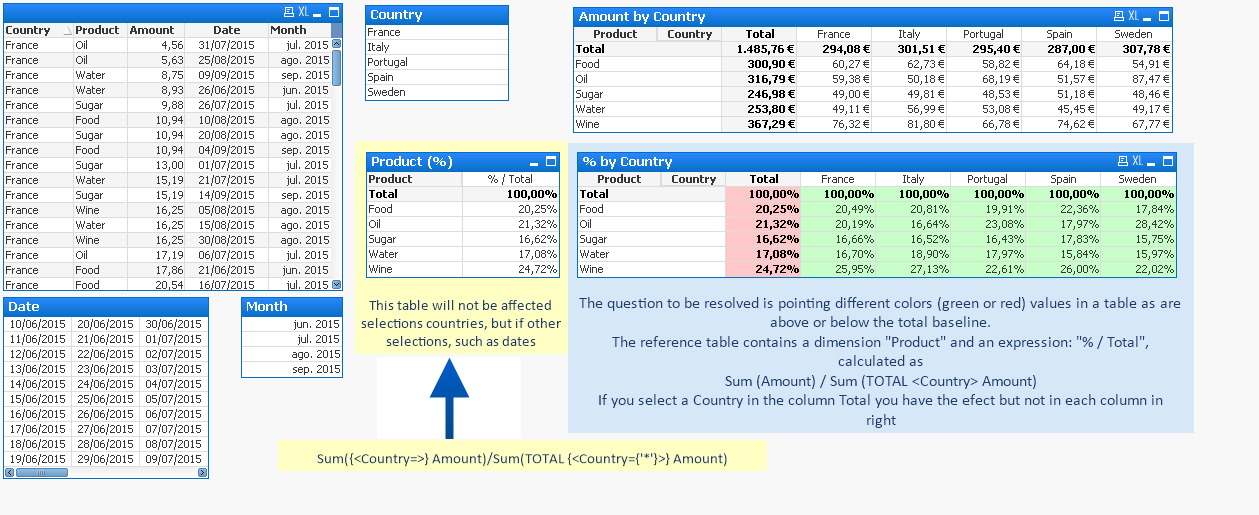# QlikView Layout & Visualizations

Discussion Board for collaboration on QlikView Layout & Visualizations.

Highlighted
Honored Contributor II

## Different colors according to value

In the example:

The question to be resolved is pointing different colors (green or red) values in a table as are above or below the total baseline.

The reference table contains a dimension "Product" and an expression: "% / Total", calculated as

Sum (Amount) / Sum (TOTAL <Country> Amount)

If you select a Country in the column Total you have the efect but not in each column in rightAnd when you select a country, the efect over total column is ok, but not over the columnsTx

Tags (2)
1 Solution

Accepted SolutionsMVP

## Re: Different colors according to value

Hi Enrique, try with this color expression:

if(Sum(Amount)/Sum(TOTAL <Country> Amount) >

Sum(TOTAL <Product> {<Country=>} Amount)/Sum(TOTAL {<Country={'*'}>} Amount), rgb(200,255,200), rgb(255,200,200))

Btw, when there are no countries selected, all totals are in red because the comparion in the if is equal ('='), so it's not '>' and goes to the else.

2 RepliesMVP

## Re: Different colors according to value

Hi Enrique, try with this color expression:

if(Sum(Amount)/Sum(TOTAL <Country> Amount) >

Sum(TOTAL <Product> {<Country=>} Amount)/Sum(TOTAL {<Country={'*'}>} Amount), rgb(200,255,200), rgb(255,200,200))

Btw, when there are no countries selected, all totals are in red because the comparion in the if is equal ('='), so it's not '>' and goes to the else.Partner